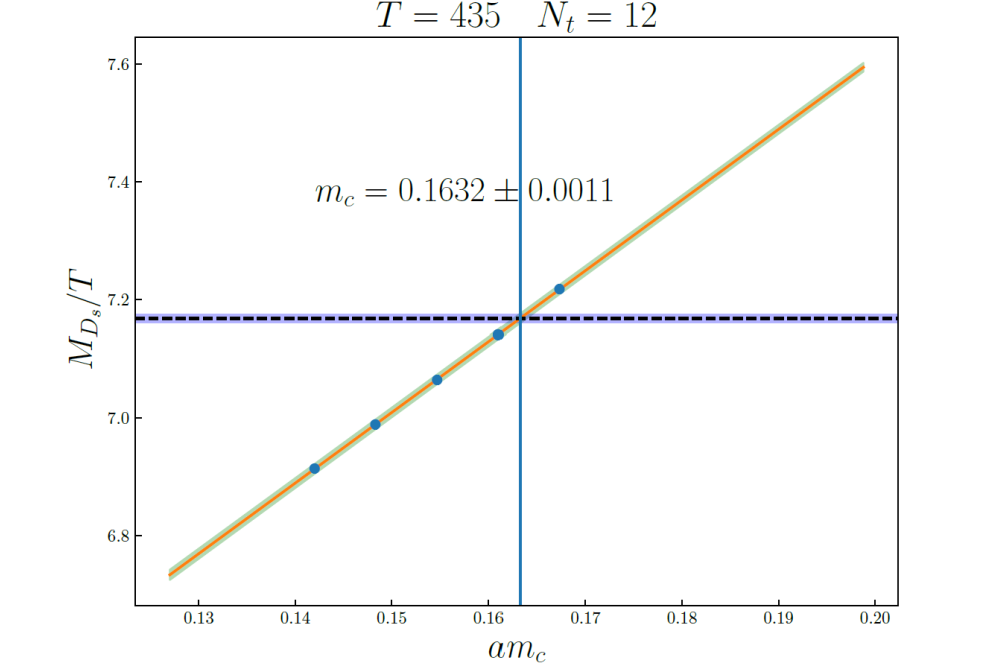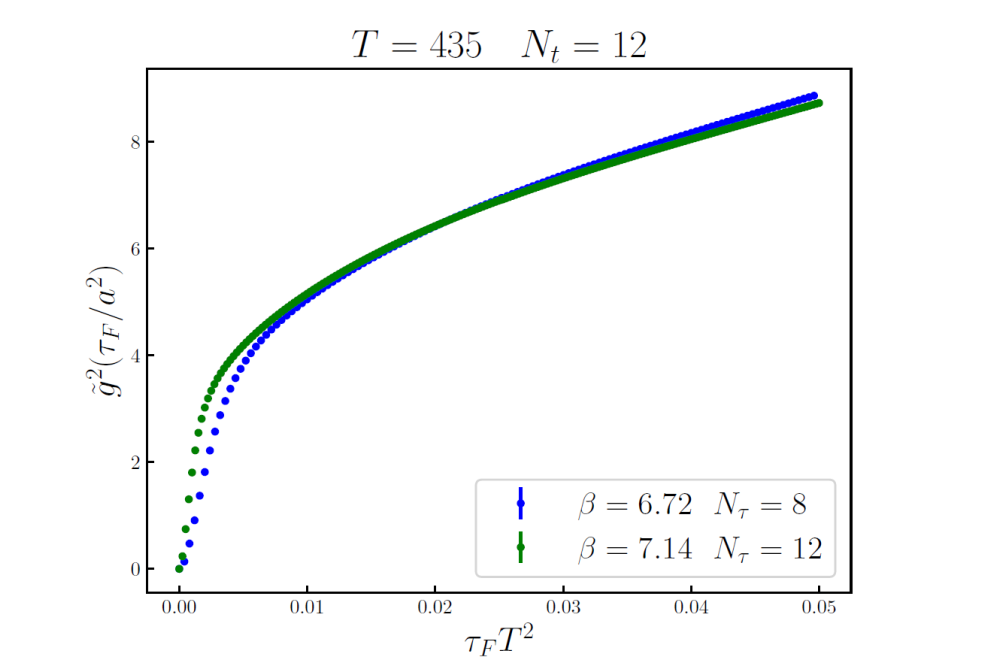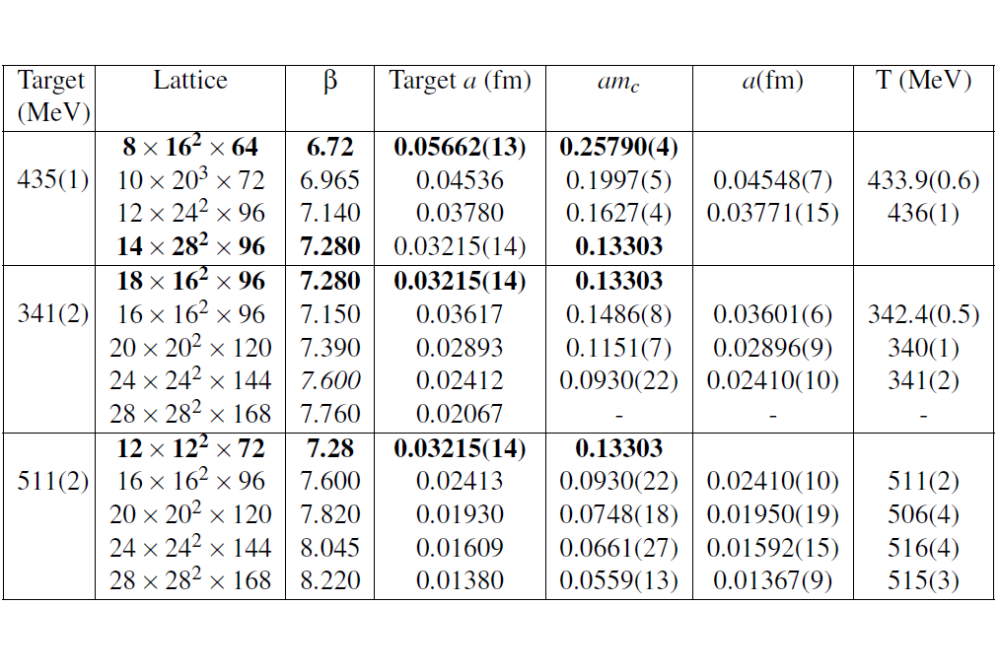# Precision Scale Setting Directly at High Temperatures Using Gradient FlowJunnarkar_Parikshit_Topological Susceptibility at High temperatures from Lattice Quantum Chromodynamics_Figure1

Figure 1: Charm quark mass at temperature 435 MeV.

Parikshit M. JunnarkarJunnarkar_Parikshit_Topological Susceptibility at High temperatures from Lattice Quantum Chromodynamics_Figure3

Figure 2: Tuning of gauge coupling at temperature 435 MeV.

Parikshit M. JunnarkarJunnarkar_Parikshit_Topological Susceptibility at High temperatures from Lattice Quantum Chromodynamics_Figure2

Figure 3: Lattice parameters for the scale setting calculation. Values in bold are known from [4,5]; Other parameters are final results of the calculation.

Parikshit M. Junnarkar

## Introduction

The overarching goal of our research project is the determination of the QCD topological susceptibility Xtop(T) at very high temperatures where it becomes relevant for Axion phenomenology. The non-perturbative calculation of Xtop(T) at high temperatures has numerous challenges. In this proposal we will address two of the main challenges. First one is the precise determination of temperature at lattice spacings around ≈ 0.01 fm as well as the quark masses at these temperatures. This has been termed as the scale-setting procedure. The second issue is the determination of the overlap on to an unphysical quark mass from which an extrapolation to lighter quark mass could be done. In the past allocation, we have addressed these two issues where the scale setting procedure which was dealt in work package i and is complete. Further in addition to the proposed research where it was proposed to perform scale setting at two temperatures, we have accomplished scale setting at three temperatures each at four lattice spacing. There is one lattice spacing at the third temperature which is yet to be complete which we will propose to finish in a separate small allocation. The work on the second issue of determining the overlap is partially complete and will be included in the small allocation.

## Methods

We describe here the methods used in work package i and ii. The main task of work package i was tuning the bare parameters i.e. the gauge coupling and quark masses to their physical values. For tuning the gauge coupling we have used the technique of Gradient flow  where the gauge fields are evolved with respect to a new flow parameter under a diffusion equation. In the first step, we perform the gradient flow for a bare coupling whose lattice spacing is known and denote this as the reference flow. The tuning then implies finding a new bare coupling at a finer lattice spacing such that it matches reference gradient flow. The tuning of quark masses is performed after tuning the gauge coupling. In our set up we have used four flavors of quarks, the heaviest being the charm quark. The tuning of charm quark mass proceeds by first computing the screening mass of the Ds meson at known charm and strange quark masses. We then use the initial guess of the charm quark mass to choose five candidate quark masses and compute the screening masses. The matching is then done by fitting these to the known screening mass.

## Results

The results of the tuning of the gauge coupling and quark masses are shown in Figure 2 in the left and right panels respectively. In both figures the tuning was done for the temperature of 435 MeV and at a lattice spacing of ≈ 0.0376 fm. The result in the left panel shows the evolution of the gauge coupling under gradient flow at two different bare gauge couplings and temporal extents. The data in blue is the reference flow i.e, the gradient flow for the bare gauge coupling which was already tuned by the MILC collaboration . The tuning of bare coupling at the same temperature for lattice with temporal extent t/a = 12 was found to be at β = 7.14. This is shown in data in green where the matching to the reference flow can be seen up to lattice artefacts and thermal effects both of which are included in the final fitting. The tuning of the charm quark is shown in the right panel of the Figure 2. The data for Ds mesons are shown in blue points in the right panel and the horizontal blue line is the reference Ds meson computed from known quark masses tuned by the MILC collaboration. The green line is the fit to the data points and the intersection yields the tuned charm quark for the temporal extent t/a = 12.

## Discussion

With the computer allocation in the period of Dec 2020 - Dec 2021, we have performed scale setting calculations at three temperatures with four lattice spacings each. We have introduced new methodology for tuning the gauge coupling and quark masses and have shown that it works successfully. This is evident in the percent level determination of the lattice spacings at different temperatures as shown in Table. We would also like to emphasize that that we added one more temperature to the overall scale setting than what we had originally proposed and that this was accomplished with modest use of resources. Due to this, the work packages ii and iii were partially completed. In particular, for the work package i, there is one lattice spacing where the scale setting procedure is partially completed and we will need more resources to complete this work package. In addition, the computation determinant ratio for quark mass reweighting also remains to be determined. We believe that all of the above can be completed within a small project allocation.

## Last Update

• Last Update: 2022-06-17 16:04